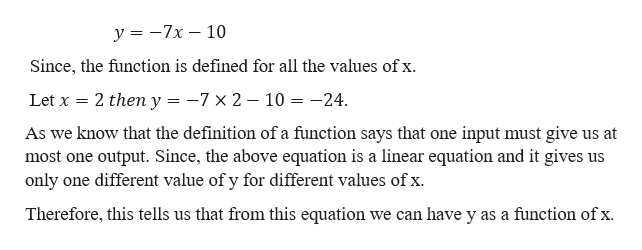# Determine whether the following equation defines y as a function of x.y=-7x-10

Question
23 views
Determine whether the following equation defines y as a function of x.
y=-7x-10
check_circle

Step 1

Given,

...help_outlineImage Transcriptionclosey-7x - 10 Since, the function is defined for all the values of x Let x 2 then y = -7 x 2 - 10 = -24 As we know that the definition of a function says that one input must give us at most one output. Since, the above equation is a linear equation and it gives us only one different value of y for different values of x. Therefore, this tells us that from this equation we can have y as a function of x fullscreen

### Want to see the full answer?

See Solution

#### Want to see this answer and more?

Solutions are written by subject experts who are available 24/7. Questions are typically answered within 1 hour.*

See Solution
*Response times may vary by subject and question.
Tagged in
MathAlgebra

### Other